# 3-D Magnetic Data Inversion with Physical Bound

3752 words (15 pages) Essay in Engineering

30/08/17 Engineering Reference this

Disclaimer: This work has been submitted by a student. This is not an example of the work produced by our Essay Writing Service. You can view samples of our professional work here.

Any opinions, findings, conclusions or recommendations expressed in this material are those of the authors and do not necessarily reflect the views of UK Essays.

A new method

Mohammad Rezaie1*, Sahar Moazam2

1 PhD in mineral exploration, Faculty of Mining, Petroleum and Geophysics, Shahrood University of Technology, Shahrood, Iran.

2 MSc in mineral exploration, Faculty of Mining, Petroleum and Geophysics, Shahrood University of Technology, Shahrood, Iran.

Abstract

Inversion of magnetic data is an important step in the interpretation of practical data. Smooth inversion is a common technique for inversion of magnetic data. Physical bound constraints can improve the solution of the magnetic inverse problem. However, how to introduce the bound constraint into the inversion procedure is important. Imposing bound constraints makes magnetic data inversion a non-linear inverse problem. In this study, a new algorithm for 3D inversion of magnetic data is developed which use an efficient penalization function for imposing bound constraints and Gauss Newton method to achieve the solution. An adaptive regularization method is used for choosing regularization parameter in this inversion approach. The inversion results of synthetic data show that the new method can produce models which adequately match with real location and shape of synthetic bodies. The test carried out on the field data from Mt. Milligan Cu-Au porphyry deposit shows that the new inversion approach could produce the magnetic susceptibility models consistent with the true structures.

Keywords: Magnetic data, Inversion, physical bound, Gauss Newton, Regularization.

1. Introduction

Magnetic surveys can provide useful information about the Earth’s interior. Magnetic measurements are usually used to delineate magnetic anomalous bodies and indicate their locations and depths. One of the most important topics in the quantitative interpretation of potential field data is the inversion of practical data (Rezaie et al. 2015). Inversion can be defined as a mathematical procedure that constructs a subsurface property (susceptibility) model using measured (magnetic) data by incorporating a priori information as available. The recovered models must predict the measured data adequately (Foks et al. 2014). 3D inversion of potential field data such as magnetic data is generally difficult (Jin et al. 2013). The main difficulty is the non-uniqueness of the solution in magnetic inverse problem. There are infinite equivalent source distributions that produce the same measured magnetic data set (Blakely 1996). The standard approach to overcome this issue is applying a priori information. Several approaches have been introduced for incorporating priori information into the inversion process (Last and Kubik 1983; Barbosa and Silva 1994; Li and Oldenburg 1996, 1998, 2003; Pilkington 1997, 2008; Portniaguine and Zhdanov 1999; Farquharson 2008; Lelievre et al. 2009; Zhang et al. 2015).

Last and Kubik (1983) developed the compact inversion method which produce compact and structurally simple model. Guillen and Menichetti (1984) minimize the moment of inertia of the body with respect to the center of the body or along single axis passing through it. Barbosa and Silva (1994) generalize the moment of inertia functional to impose compactness along several axes. Li and Oldenburg (1996, 1998) developed a model objective function that produce smooth models. This method can locate anomaly sources accurately nevertheless, the values of the recovered model are smaller than the true values due to the smoothness effect of the objective function. Portniaguine and Zhdanov (1999) developed a focusing inversion method based on compact inversion method for potential field data. Barbosa and Silva (2006) developed an interactive method for inverting magnetic data with interfering anomalies produced by multiple, complex, and closely separated geologic sources. Farquharson (2008)
used L1 measure of Li and Oldenburg’s model objective function to recover dipping structures and models which have angled interfaces.  Lelievre et al. (2009) used Li and Oldenburg’s (1996, 1998) model objective function and developed advanced constrained inversion by geological information. Zhang et al. (2015) improved Li and Oldenburg’s method by applying Lagrangian multipliers in the model objective function to add geological constraints. In the 3D inversion of potential field data, particular bounds of the physical property may be known. This physical bound constraint can improve the solution and make it more feasible (Rezaie et al. 2017a). Consequently, how to introduce the bound constraint into the inversion procedure becomes an important issue. Portniaguine and Zhdanov (1999, 2002) used a penalization algorithm to impose bound constraint in focusing inversion of potential field data. Li and Oldenburg (2003) chose a logarithmic barrier method incorporating bound constraints on the recovered smooth model. Zhang et al. (2015) imposed bound constraint in smooth inversion of potential field data via a method using Lagrangian multipliers. However, imposing bound constraint makes magnetic data inversion a non-linear inverse problem. Therefore, the logarithmic barrier and Lagrangian multipliers methods increase computation time. Another issue in solving non-linear inverse problem is choosing regularization parameter that can increase computation time (Farquharson and Oldenburg, 2004).

In this study, we develop a new 3D magnetic data inversion method based on the Gauss- Newton (GN) algorithm that can incorporate bound constraints on the recovered model using penalization algorithm introduced by Portniaguine and Zhdanov (1999, 2002). Also, we will use an adaptive regularization method for regularization parameter selection in our magnetic data inversion method. Finally, the capabilities of the proposed method are illustrated by its application to the inversion of a synthetic data set and to the 3D inversion of magnetic data from the Mt. Milligan deposit at British Columbia, Canada.

2. Methodology

2.1. Forward model for 3D magnetic anomalies

Susceptibility distribution in the sub-surface (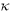) produce magnetic field (T) at the surface. The purpose of forward modeling is to compute this magnetic field. The total component of the magnetic field is given by (Blakely 1995):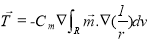(1)

where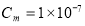(Henry.meter-1), R denotes the volume occupied by causative body.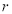is distance and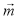is magnetization vector which can be obtained as a vector sum: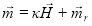(2)

where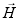is earth’s magnetic field and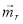is remanent magnetization. If we ignore remanent component, the magnetization will be in the direction of the earth’s field and can be obtained simply as: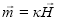(3)

To compute total component of the magnetic field in Eq. (1), it is required to discretize the subsurface under the survey area into rectangular prisms of known sizes and positions with constant susceptibilities. The formulation for computation of magnetic response for each rectangular prism was presented by Bhattacharyya (1964) and later simplified into a form that is more suitable for fast computer implementation (Rao and Babu 1991). We use the formulation developed by Rao and Babu (1991) to compute magnetic response resulting from individual prisms. If the observed magnetic anomalies are caused by M subsurface prisms, the magnetic field at the field point i is given by: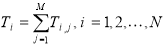(4)

where N is the number of observation point. The forward modeling of magnetic data using Eq. (1) and Eq. (4) can be written as following matrix equation: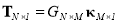(5)

Here, G is forward operator matrix that maps the physical parameters space into the data space.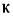denotes the vector of unknown model parameters and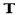is data vector that is given by measurement data. There are some error in measurement data because of noise that is usually assumed to be uncorrelated and have Gaussian distribution (Rezaie et al. 2017b), So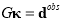(6)

where,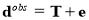is vector of observed data and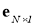is vector of data error. The main purpose of the magnetic inverse problem is to find a geologically plausible susceptibility model ()based on G and some measured data (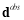) at the noise level.

2.2. Inversion method

In the typical minimum-structure inversion procedure, subsurface of the survey area is discretized into rectangular prisms (cells) of known sizes and positions with the values of the physical property (e.g. susceptibility) in the cells that are called the model parameters to be estimated in the inversion (Rezaie et al. 2015). The solution can be obtained by minimization of an objective function, which is a combination of a measure of misfit between observation and predicted data and a measure of complexity of the model subject to a physical bound constraint (Li and Oldenburg 1996):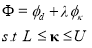(7)

where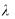is a regularization parameter. L is lower susceptibility bound, U is the upper susceptibility bound and the misfit functional is defined as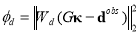(8)

Here,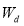is data weighting matrix given by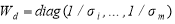. Where,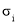stands for the standard deviation of the noise in the ith datum, and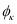is a stabilizing functional (stabilizer) which measure minimum norm of model structure (Li and Oldenburg 1996, 1998, 2003):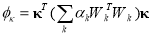(9)

where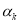are coefficients that affect the relative importance of derivative components in different directions.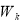resembles first-order finite-difference matrices in x, y and z directions. We have to use an additional depth weighting matrix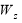for compensating lack of the data sensitivity to the deeper model parameters (Zhdanov 2015):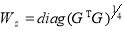(10)

Now, Eq. (9) can be reformulated to apply the depth weighting matrix to the objective function.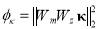(11)

where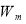is the cumulative first-order finite-difference matrix. Eq. (7) is reformulated using matrix notation to incorporate depth weighting easily: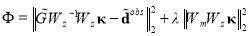(12)

where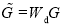and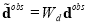. Eq. (12) is transformed into a space of weighted model parameters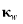by replacing the variables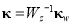and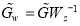(Rezaie et al. 2017a):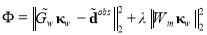(13)

The solution of Eq. (13) is obtained according to the regularization theory similar to the classical minimum norm optimization problem (Tikhonov et al. 1977). The solution of the magnetic inverse problem is obtained by minimizing this equation using the GN method. The upper (U) and lower (L) susceptibility bounds can be imposed during the inversion process to recover more feasible model. If an achieved susceptibility value falls outside the bounds, the value at that cell is projected back to the nearest upper or lower susceptibility bound (Portniaguine and Zhdanov 1999).

To solve Eq. (13) with GN method, assume the obtained solution denoted by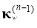at the (n − 1)th iteration, and the predicted data corresponding to this model are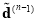.Then at the nth iteration, a model perturbation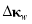can be achieved by solving following equation so that the inverted model can be updated by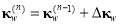(Aster et al. 2013):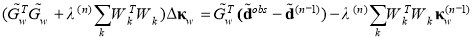(14)

where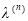is the regularization parameter in nth iteration. Then the solution of the inverse problem in Eq. (7),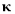is given by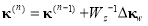(15)

In order to recover a more feasible model of the subsurface, upper (U) and lower (L) physical bounds of susceptibility are imposed in each iteration to force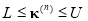. If a given susceptibility value falls outside the bounds, the susceptibility value of that cell is projected back to the nearest physical bound value.

The solution to Eq. (14) is also equivalent to the least-squares solution of(16)

The least-squares solution of the Eq. (16) is obtained by a fast iterative method such as Lanczos Bidiagonalization (LB) (Pagie and Saunders 1982) at each GN iteration. therefore, the proposed algorithm would be suitable for large scale problems (Rezaie et al. 2017a,b). The GN iterations stop when the RMS misfit reaches an acceptable level or the model corrections become small enough (Pilkington 2008).

We have used an adaptive method for choosing regularization parameter similar to which was proposed by Farquharson (2008) which is a fast and efficient algorithm for choosing regularization parameter. The regularization parameter is started at 100 (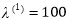) which is a relatively large value. If an inversion is performed with the regularization parameter fixed at this value, a model would be produced that had a small amount of structure and predicted data under fit the observations. At each iteration, the regularization parameter is damped to give a slow but steady progression of models with increasing structure and decreasing data misfits: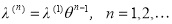(17)

where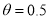based on empirical experiments.

3. Synthetic test

We apply our algorithm to a synthetic test to evaluate the reliability of the introduced method. The synthetic model consists of two different blocks with dimension 200 m – 200 m – 200 m which are embedded beneath the surface so that susceptibility of uniform background is zero. The Susceptibility of each block is 0.06 (SI). Perspective view of the true model is displayed in Fig. (1a).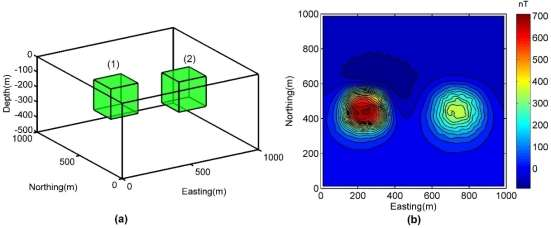Fig. 1 perspective view of the synthetic model with 2 blocks (a). Magnetic anomaly produced by the synthetic model with 5 % Gaussian noise of the accurate datum magnitude.

Depth to the top of the shallower block (block (1)) is 50 m and depth to the top of the deeper block (block (2)) is 100 m. The total-field anomaly data have been generated above the surface assuming an inducing field with inclination (I) of 75, declination (D) of 25 and a strength of 50000 nT. The data generated over a grid of 1000 m – 1000 m with sample spacing of 25 m. There are 1600 data and 5 % Gaussian noise of the accurate datum magnitude has been added (Fig. 1b). The subsurface is divided into 40 – 40 – 20 = 32000 rectangular prisms with the same size of 25 m for inversion. The inverse problem has been solved using the proposed method that is described in the preceding section (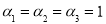). The solution obtained after 5 iterations with RMS of 0.05.

Fig. 2 shows a plan section and a cross section through the recovered model from proposed inversion method. The result indicates acceptable smooth reconstruction of the synthetic multisource blocks at different depth levels below the surface. The recovered bodies in the model are smooth and adequately matched with real location of synthetic bodies.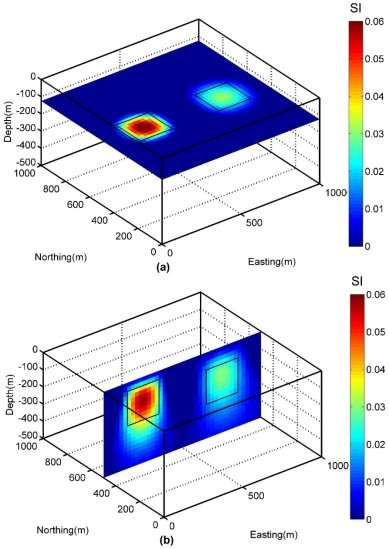Fig. 2 Plan sections through the recovered susceptibility model obtained from the 3D inversion of magnetic data at depth= -125 m (a). A cross-sectional slice of the susceptibility model at Northing= 500 m (b). The borders indicate the true position of each body.

4. Inversion of field data

Mt. Milligan is a Cu-Au porphyry deposit situated in central British Columbia. Geological information obtained from a major drilling program show the host rocks of the deposit are Mesozoic volcanic and sedimentary rocks and contain intrusive monzonitic rocks that have accessory magnetite. There is an intensive hydrothermal alteration primarily in the region near the boundaries of the monzonite stock. The monzonite body is known as the MBX stock (Oldenburg et al. 1997). The copper and gold are concentrated in the potassic alteration zone, which is mainly around the contact of the monzonite intrusions (MBX) and may extend outward and into fractured volcanic rocks. However, magnetite is one of the strong indicators of the potassic alteration. In this region, magnetic data are acquired at 12.5 m spacing along lines in the east direction that spaced 50 m apart (Li and Oldenburg 1996). We use the data at 25 m spacing which yields 1920 data points. The reduced magnetic anomaly map is shown in Fig. (3).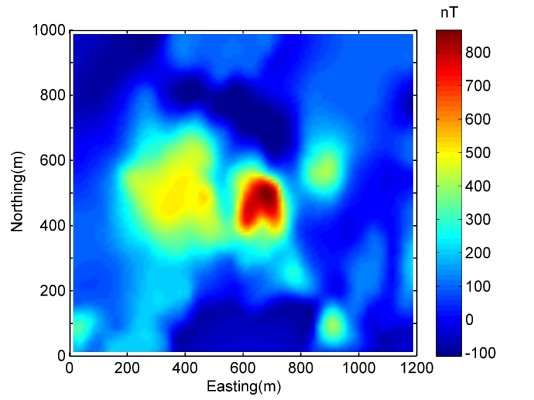Fig. 3 The magnetic anomaly map of Mt. Milligan. The data are on 25 m – 25 m grid.

The direction of the inducing field is I= 75 and D= 25.73 with a strength of 58193 nT. It is assumed that each datum have an error whose standard deviation is equal to 5 percent of its magnitude (Li and Oldenburg 2003).

To invert these data, the subsurface of the area is discretized into 48 – 40 – 18 = 34560 cells each of size 25 m. The positivity constraint was imposed which means lower (L) physical bounds of susceptibility are set to 0 SI. The solution is obtained after 112 iteration with RMS error of 0.05 which is about the predicted noise of the data. The recovered model is shown in Fig. (4) as one plan-section and one cross-section. The true edge of MBX stock and mineral assemblage which were derived from the drilling results overlaid on the cross-section.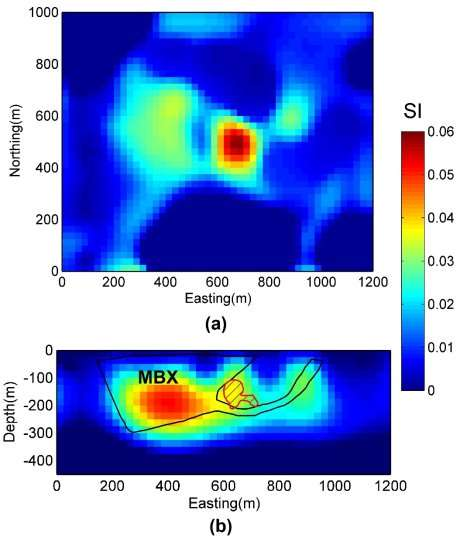Fig. 4 The recovered susceptibility model shown in a plan-section at the depth of -80 m (a). A cross-section at the northing of 600 m overlaid by true boundary of monzonite body (MBX) with black line and mineral deposit with red shaded polygon (b).

The results indicate that the anomalous body of magnetic susceptibility highs are mostly associated with the monzonite intrusion. There is a moderate anomalous body at the center of cross-section which is probably caused by magnetite content of potassic alteration. This area coincides with mineral deposit. Thus, the obtained solution is in a good agreement with true geologic boundaries of Mt. Milligan deposit.

5. Conclusions

We have developed a new algorithm for inversion of magnetic data using Gauss Newton method. In each GN iteration LB method is used for solving least- square problem. Therefore, the proposed algorithm is efficient for large scale problems. We used an adaptive regularization method for choosing regularization parameter in each iteration which is a fast and efficient method for choosing regularization parameter. In the new algorithm, the physical bound constraint can be imposed during the inversion process via penalization function which does not need any transformation. Therefore, this method of imposing bound constraint is more efficient.

The obtained results show the new developed 3D inversion method is able to produce a smooth solution which define the shape and extent of synthetic bodies adequately. Furthermore, the application of this inversion algorithm for a field magnetic data from Mt. Milligan deposit produced a model that is consistent with the available geological information.

Compression methods such as wavelet compression which can compress the kernel matrix and using parallel programing that decrease the required memory and computation time will be subject of future works for large scale problems.

#### Cite This Work

To export a reference to this article please select a referencing stye below:

## Related Services

View all

### DMCA / Removal Request

If you are the original writer of this essay and no longer wish to have the essay published on the UK Essays website then please:Prices from
###### £28

Undergraduate 2:2 • 250 words • 7 day delivery

Delivered on-time or your money back

##### Rated 4.6 out of 5 by(146 Reviews)